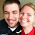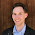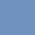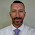## Pic## Sunday, May 4, 2014

### Exponentiation Is Like Place Value, Unlike Addition

You get two digits: "2" and "3," and you get to use them only once. Using the language that appears in school math and these two digits, how many different numbers can you denote?

Here are 16 ways that I came up with to do something with those two digits.

It seems sort of silly to group all of these together. I mean, "32" is an entirely different sort of answer than "2+3."

Let's distinguish between two categories. On the one hand you have the operations, the things that perform some sort of process (or mapping) on two different numbers that yields some third number.

I think that adding, subtracting, multiplying and dividing are all clearly examples of performing some operation on 2 and 3. So all of these go into my "operation" pile.

It really seems to me that "23" and "32" don't belong in this pile. I did some other trick with my starting digits to get 23 and 32. I didn't really perform some process on the digits 2 and 3 to produce a third number. Instead, I used those digits to express a new number. It was just smushing the digits together -- I wasn't combining them with some operation like addition or multiplication.*

* Math Major sez: Oh really? You just performed the mapping (a,b) --> (10a + b). Yeah right, Math Major, yeah right.

This second trick of mine is more about using those starting digits two express a new number. I'm not even treating my starting digits as numbers in their own right, not really. What I'm really doing is treating them like letters of the numerical Alphabet, and lining them up to "spell" some number.

It's obvious to me that "23" and "32" were produced by "spelling" a new number with my starting digits. I think that I also did this with the decimal point.

I've got two categories now: the results of performing some process on numbers, and the results of expressing some number with digits.

This leaves 6 of my 16 results uncategorized.

Next up, I want to tackle the fractions. Some subtlety is needed here, because you can see the fraction bar as a division symbol and it wouldn't be a mathematical sin. It's a bit of a fielder's choice, in that sense. Still, I think of fractions as numbers in their own right, not some sort of quotient waiting to be revealed. I'm going to sort them into "numbers that I expressed" instead of "numbers that I got through performing an operation."

That leaves radicals and exponents. And it's the exponents that I'm really interested in.
If you think that "2 to the third power" means something like "multiply 2 by itself 3 times," then I think that this number pretty obviously goes in the "result of an operation" pile.

The alternative, though, is to think of "2 to the third power" as quite a great deal like "23." It's not so much the result of operating on the numbers 2 and 3, as much as it is using those digits to express some number.

So, which pile does it go in?

Christopher Danielson has pointed out that there is no really good way to talk about the "result" of exponentiation. For addition you get the sum, for division you get the quotient, but how do you talk about what you get out of exponentiation?

I take Danielson's observation as partial evidence that our number language has stacked the deck against thinking of exponentiation as a operation. Instead, the way we talk about exponentiation suggests that it's best seen on the model of "23" or "32," as using digits to express some number.

If this is true, then it partially diagnoses the major problems that students have in learning about exponents. Their teachers have been teaching exponentiation as a process, when it's really a feature of the expressive power of mathematical language.

1.Interesting thoughts! These are great things to puzzle about!

2.So what about that square root? My perception is that sqrt(3) is the expression of a new number, while sqrt(16) is an unfinished operation.

I actually touched briefly on this with the students last week. We discussed how (3/2)*36 'means' three half-36s (speaking additively), and compared that with 36^(3/2) meaning three half-36s (speaking multiplicatively). I can see a more thought-out approach treating the symbols 16 as being an additive-number notation: ten plus one plus one plus one plus one plus one plus one, and 4^2 as being a multiplicative-number notation: four times four.

That prompted another thought for me: prime factorization. Don't we explain the prime factorization as *being* the number, not just a string of operations that reduce to the number?

3.Thanks for the thoughtful breakdown of this challenging topic to communicate.

Perhaps the challenge is that exponentiation is both the operation and the value. 2^3 can be seen as equivalent to the value of 8 or as a notation/representation of the operation 2x2x2 which has an equivalent value. If exponentiation is the value, then by equivalence/transitive property it must also be the operation - they are the same. The value of the operation can be understood through the pattern of repeated multiplication/division of a particular number.

I talk to my classes about the notation/representation of the operation and the equivalence to a value. I don't think seeing it as the value needs to exclude seeing it as a representation. We talk about the fact that 16/2, 2x2x2, sqrt(64), 6+2, and 2^3 all represent the same value and therefore are equally suitable representations of all operations or expressions that have the same value.

Don't know if this adds anything to the discussion, but want to argue that it need not fall on one side of a fence all the time in order for it to be understood.

1."Perhaps the challenge is that exponentiation is both the operation and the value. 2^3 can be seen as equivalent to the value of 8..."

Two thoughts:

1. 2^3 isn't significantly different than 23. You can think of 23 as the result of some operation (2*10 + 3) as well, so it's not about what ways are possible to see exponentiation, but what ways are natural.

2. To say that 2^3 can be seen as equivalent to the value of 8 is, to my mind, just another way of using the operation framework.

What I'm saying is that seeing 2^3 as 8 is kind of like seeing 10 as IIIIIIIIII.

3. With fractions, we don't start off telling our students that 2/4 means "divide 2 by 4." Instead, we work hard to make sure that they understand 2/4 as an actual number, and then later they develop the relational view that fractions can be seen as division. I suspect that a similar process would be helpful for students learning exponentiation.

2.In Extending Children's Mathematics, the CGI fractions book, they do argue for introducing 2/4 as division first. 4 kids share 2 cookies fairly. How many cookies does each kid get? builds a meaningful understanding of both fractions and division.

3.I think that there's a difference between CGI recommends and what I'm talking about. CGI doesn't say that you should introduce a 1/3 as "one divided by three." Instead, they say that it's the number that represents the amount that you get when you solve a division problem.

Similarly, I'd say that 2^3 represents a number that you get when you solve a problem such as "What's the third power of 2?" Or "What's 2 groups of 2 groups of 2?"

The way that we typically introduce exponents would be like skipping straight to the relational understanding of fractions, in CGI.

4.Unless I've gotten all this wrong.

Side note: I tend to wildly swing between extreme confidence and extreme doubt. I've been thinking about this for a while, but I also know that I always screw up. I find the adversarial back-and-forth helpful, but I know that can come off as a lot of bluster. Hopefully I'm able to find the balance in my writing, but it's something I struggle with.

5.CGI says to introduce the concept of equal sharing to build background knowledge for the concept of a fraction. They feel that fractions represent a great number of meanings: n parts out of m parts, the amount of stuff- 2/3s of a bar, relationships of amounts- 2 dollars for every 3 people, process of amounts- 2 dollars shared among 3 people. They can also be the mathematical representations we currently view them as.

Overall I would place fractions as the result of an event. Technically does that place it as an operation? To us it may represent a number, but that is a comparison to a whole. Do fractions happen naturally or are they a result of our mathematical manipulations?

I am also sad to see no trig functions here or logs....

6.Michael, you're coming off thoughtful and open, so no worries. :) You're much better at precision of mathematical language than I am, and more inclined to want to nail down the categories. I tend to dig the duality (or multipality?) of different mathematical ideas. For example, zero is both nothing, and something. Groovy. I like that. So, I'm inclined toward thinktoaction's thoughts about being both an operation and a value. Which one we're referring to in any given instance depends on the context of the conversation, no? I'm thinking particularly about the very different visual images that pop into my mind when I think about cubing units to describe volume vs. how many pieces of paper I'll have when I cut a piece of paper in half, and half again, and half again.

And I've never given much thought to groups of groups of groups. Thank you for that! I need to chew on that one for a while.

7."I tend to dig the duality (or multipality?) of different mathematical ideas."

Right! On twitter, Malke Rosenfeld sent us to Anna Sfard's article on process/object duality. Duality is really interesting.

But. But there are pedagogical questions to consider. For instance, multiplication can be seen as repeated addition, and it can also be seen as a "number of groups" operation. There's (at least!) duality here, but many people think that it's more productive to begin with one particular conception.

So: which conception of exponentiation is most helpful for beginners to exponents?

8.Oooh, I'll read it when I get home. Fun! So, you're asking a great question. I've done zero thinking about the best conception of exponentiation from a pedagogical point of view, given that the highest grade I've taught was 4th. For me, personally, I think I first NEEDED exponents when I needed to describe something that was growing shockingly fast in a way I'd never seen before. Something like population explosions created a need for a new way to describe a different kind of growth. And that's where order of operations started to make sense. Exponential growth just kicks multiplicative scaling to the curb, and multiplication makes addition seem like really small potatoes.

I was taught exponents and rules of exponents in the same boring way everyone else was taught. It wasn't until I saw how they could--in just a few numerals--describe something getting so big so fast that I actually cared about them. I'd seen the power of powers, so to speak. Har de har har.

9.One more thought, though. I think we sometimes overthink the sequencing/compartmentalization of concepts. The unintentional but unfortunate consequence is students end up with compartmentalized knowledge, and fail to make the connections between ideas. If you asked some 8th graders to write EXPONENTS in the middle of a piece of paper and make a semantic map, what would they write? Are they seeing that this rule they learned in 6th grade and this volume they learned about in 5th grade and these graphs they made in 8th grade, all connect and relate? Or did we sequence the connections right out of their learning?

4.I think this would be a really interesting activity to have students at different ages complete. Give them the categories with a short explanation and then have them sort the different representations. It may lead to some misconceptions about what they (the representations) really mean.

Great discussion of something that in my mind at first was very simple!

5.I am loving this discussion because I think about these things a lot, too.
One of the reasons CGI emphasizes the fair share/division model of fractions so much is because student typically get instruction so biased to part/whole that they have a hard time later recognizing fractions as division statements, as numbers, or as ratios later on. It might be better to call the "division" they do "partitioning" instead. It seems more in keeping with the actions than the operation of division, with all of its abstract algorithm baggage.

1.I think they emphasize it mostly because they watch how kids think, and partitioning is a strategy they see kids use. My understanding of CGI is they're coming at math education from a place of seeing what kids already know and do, and then think about pedagogy from there, rather than starting with what pedagogy they think we should use. Know what I mean? I don't think it's a reaction to our prior teaching. Am I wrong?

2.I take your point - that is the CGI approach to look at development first and let that guide pedagogy. I still think both are true. Some of the part-whole has to be moderated in order for kids to make sense of improper fractions and the other models.

3.Absolutely.

6.Here are some quotes from _Where Mathematics Comes From_ (by Lakoff & Nunez), starting on page 399:

"In general, raising a number to a power cannot be understood as self-multiplication. What then is raising a number to a power? We can see the answer most easily if we first look at logarithms. Think... of how a slide rule words. If you want to multiply two numbers, you add their lengths on the slide rule.... The reason that works is that they use a logarithmic scale: Multiplication is adding the logarithms... What is a logarithm then? A logarithm is a mapping that allows you to multiply by adding.... Let us look at some of its properties. It maps real numbers onto real numbers and products onto sums. That is, it maps a product a * b onto a sum a' + b' by mapping numbers a and b onto a' and b' and by mapping the operation of multiplication onto the operation of addition.... Log is a mapping from the group of positive real numbers with the operation of multiplication to the group of all real numbers with the operation of addition. As such, log maps the multiplicative identity, 1, onto the additive identity, 0."

7.It goes on to give constraints of the mapping and talk about the idea of different bases. So one map has the constraints:
1 is mapped onto 0
10 is mapped onto 1
10^n is mapped onto n
Products are mapped onto sums

And in general
1 is mapped onto 0
The chose base b is mapped onto 1
b^n is mapped onto n
Products are mapped onto sums.

That's enough to uniquely define the mapping, but not enough to determine how to calculate the mapping... which is why John Napier is famous and an important contributor to math and astronomy, and why we use calculators to do almost all log problems.

So, now some exponentials quotes:
"As we have just seen, the logarithm mapping with a base b, greater than 1, maps a positive real number with the structure b^n onto a real number n. Since the logarithm mapping with base b is one-to-one, there will be an inverse mapping that maps a real number n onto a positive real number b^n. This is the exponential mapping. It is the inverse of the logarithm mapping, and its general constraints are:
0 is mapped onto 1
1 is mapped onto b, for b greater than 1
n is mapped onto b^n, for all real numbers n
Sums are mapped onto products"

"For... special cases... it so happens that they can be computer via self-multiplication. For example, 2^6 can be computed by multiplying 2 * 2 * 2 * 2 * 2 * 2 = 64. But the exponential mapping applies to an infinity of cases where this is not true.... It is important to understand the difference between self-multiplication and exponentiation. When we write 32 = 2 * 2 * 2 * 2 * 2, we are implicitly ... [understanding] 32 is a whole that is made up of five instances of the same part, 2, put together by multiplication. The symbolization 2^5 as usually understood is, from a conceptual point of view, ambiguous. It could mean "2 * 2 * 2 * 2 * 2" or it could mean "the result of the exponential mapping with base 2 applied to 5." An alternative way of writing the latter might be Exp-sub-2(5), where 5 is the input to the exponential mapping and 2 indicates what 1 maps onto in this version of the exponential mapping.... Prior to the choice of a fixed base, logarithms and exponentials are each families of functions. To choose a base -- say, 2 or 10 -- is to choose a member from the family, a unique logarithmic or exponential function."

8.And more

"The exponential function, as we have just seen, is not characterized in terms of self-multiplication; rather, the exponential is a function from the additive group of real numbers to the multiplicative group of positive real numbers. That function maps the additive identity 0 onto the multiplicative identity 1, for any choice of base b. The expression "b^0" does not mean "b multiplied by itself zero times," which makes no sense. Instead it means: "Look at the exponential function which maps 1 into b. That function also maps 0 into 1." Since this is true for any base b, it is always true that b^0 = 1.
"The way that the exponential function is written -- "b^x" -- is misleading. It is done that way for the purpose of ease of calculation, for those cases where self-multiplication will happen to allow you to calculate the values of the function. But the notation hides the relationship between b and x when you understand the exponential function as mapping from the domain of real numbers under addition to the range of positive real numbers under multiplication.
"In such a mapping, the "x" in "b^x" is in the domain of the exponential function, whereas the "b" is in the range of the exponential function. b is the number that 1 is mapped onto -- the number that picks out which particular mapping exponential mapping is being used. b thus constrains what x is mapped onto."